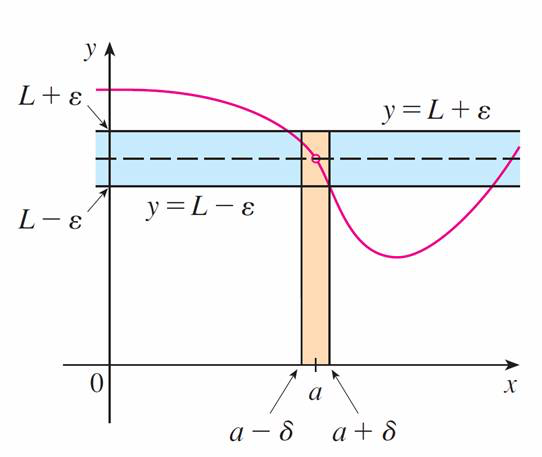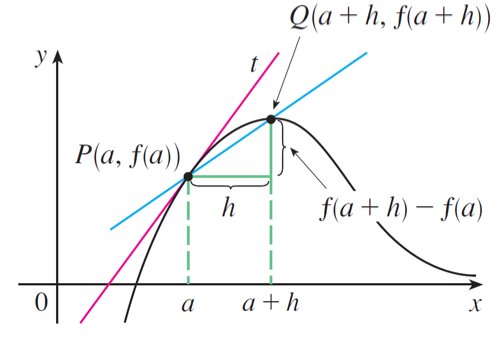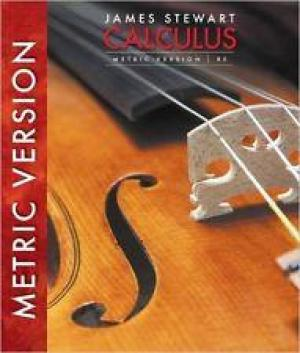# Calculus I

A core and fundamental course

## What is Calculus and used for?

The formal study of calculus started from the 17th century by well-known scientists and mathematicians Isaac Newton and Gottfried Leibniz. Calculus is a mathematical discipline that is primarily concerned with functions, limits, derivatives, integrals and infinite series. The emphasis is understanding concepts. The most fundamental concept in calculus is limit.

### The Precise Definition of a LimitLet $f(x)$ be a function defined on some open interval that contains $a$, except possibly at $a$ itself. Then we say that the limit of $f(x)$ as $a$ approaches $a$ is $L$, and we write $$\underset{x\rightarrow a}\lim f(x)=L$$ if for every number $ϵ > 0$ there is a number $δ > 0$ such that $$\text{if}\quad 0<|x-a|<\delta\quad\text{then}\quad|f(x)-L|<\epsilon$$

### Continuity

Let $f$ be a function and let $a\in\mathbb{R}$. The function $f$ is said to be continuous at the point $a$ if the following three things are true:

1. $f (a)$ is defined (that is, $a$ is in the domain of $f$.)
2. The limit $\underset{x\rightarrow a}\lim f(x)$ exists.
3. $\underset{x\rightarrow a}\lim f(x)=f(a)$

### DerivativeThe derivative of a function $f$ at a number $a$, denoted by $f'(a)$, is $$f'(a)=\underset{h\rightarrow 0}\lim\frac{f(a+h)-f(a)}{h}$$ if this limit exists.

### MIT Mathematics

Calculus is the study of how things change. It provides a framework for modeling systems in which there is change, and a way to deduce the predictions of such models.

Calculus is an exciting subject, justly considered to be one of the greatest achievements of the human intellect. I hope you will discover that it is not only useful but also intrinsically beautiful.

— James Stewart

### Reference Textbook

Calculus, James Stewart, The 8th, metric edition, Cengage Learning.